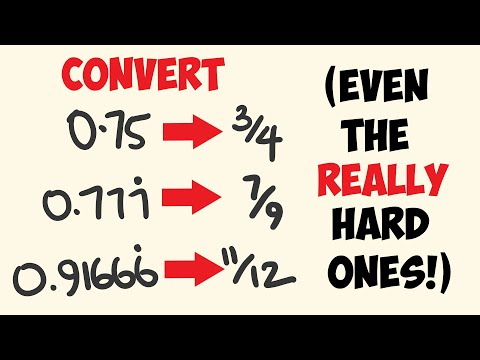Home » What is 9.6 as a Fraction? – Decimal to Fraction Conversion

# What is 9.6 as a Fraction? – Decimal to Fraction Conversion

What is 9.6 as a Fraction?

• A. 5/8
• B. 2/1
• C. 1/2
• D. 48/5
• E. 129/100

## Quick Solution:

Recognize that 9.6 equals 9 and 6/10 (since.6 represents 6 tenths).

Divide the numerator and denominator by 2, their greatest common divisor, to simplify 6/10 to 3/5.

The decimal 9.6 can then be expressed as the fraction 9 3/5.

To convert this to an improper fraction (where the numerator is greater than the denominator), multiply the whole number component (9) by the denominator of the fractional component (5) and add the numerator of the fractional component (3), which gives 45 + 3 = 48.

Therefore, the improper fraction representation of 9.6 is 48/5.

## Understanding Decimals and Fractions

Decimals and fractions are two different ways of expressing the same concept: parts of a whole. They are interrelated, and converting from one to the other helps us understand the values they represent. A decimal is a whole number plus tenths, hundredths, etc., while a fraction is a division problem: the numerator is divided by the denominator.

## Steps to Convert 9.6 as a Fraction

To convert 9.6 as a fraction, we must first separate the whole number from the decimal. So, we have the whole number 9 and the decimal 0.6. Since we know that 0.6 equals 6 tenths, we can write it as the fraction 6/10. This fraction can be made easier by dividing the numerator and the denominator by their biggest common factor, which is 2. This leaves us with 3/5. The whole number nine stays the same.

## Final Fractional Form of 9.6

9.6 can now be written as the mixed number 9 3/5. A mixed number comprises a whole number and a fraction that is the right size. Suppose we want to change this into an improper fraction where the numerator is bigger than the denominator. In that case, we can multiply the whole number part (9) by the denominator of the fractional part (5) and add the numerator of the fractional part (3). This means that 45 + 3 = 48. So, the last way to write 9.6 as a fraction is 48/5, which is an improper fraction.